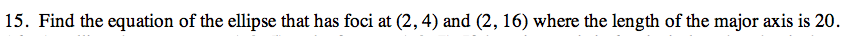13E-15.

### Missing problem for Book PCT => Chapter Ch13 => Lesson 13.1.2 => Problem 13E-15

Created from orphaned homework help problemThe center of the ellipse is located at the midpoint of the two foci.

$\left(\frac{2+2}{2},\frac{4+16}{2}\right)$